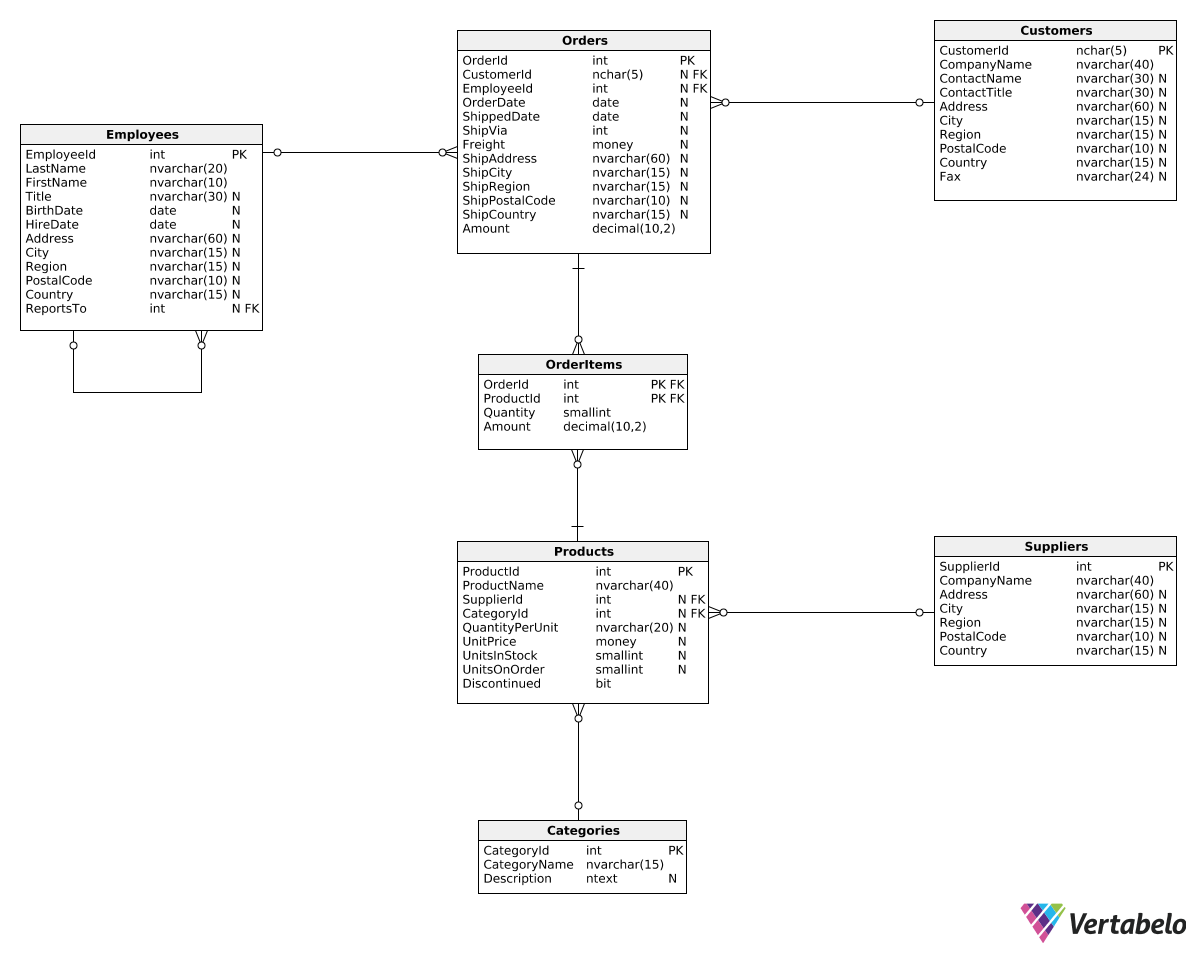Deals Of The Week - hours only!Up to 80% off on all courses and bundles.-Close
Introduction
Get to know the database
Basic revenue metrics
Summary

## Instruction

Perfect! When we wanted to find orders from March 2017, we used the following WHERE clause:

WHERE O.OrderDate >= '20170301' AND O.OrderDate < '20170401'

There is another way to write this query. Take a look:

SELECT
C.CustomerId,
CompanyName,
SUM(Amount) AS TotalRevenue
FROM Orders O
JOIN Customers C
ON O.CustomerId = C.CustomerId
WHERE O.OrderDate >= '20170301'
AND O.OrderDate < DATEADD(Month, 1, '20170301')
GROUP BY C.CustomerId,
CompanyName;


This time, instead of providing the specific end date, we used the DATEADD() function, which looks like this:

DATEADD(interval, number, date)

This function takes three arguments:

1. interval – the interval we want to add, such as year, quarter, month, or day.
2. number – the amount of that interval to add.
3. date – the date to be modified.

In our example, DATEADD(Month, 1, '20170301') means "add one month to March 1, 2017".

## Exercise

The quarter is a period of three months – the first quarter (Q1) is a period of the first three months (January, February, March), the second quarter (Q2) is a period of the second three months (April, May, June), etc. In the world of finance, the quarter is a very important time period and quarterly analyses are very common.

Show the total revenue generated in the first quarter of 2018. Group revenue by each shipping country. Show two columns: ShipCountry and TotalRevenue. Use the DATEADD() function.

### Stuck? Here's a hint!

Use the following code:

DATEADD(quarter, 1, '20180101')